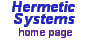Radian-Degree CalculatorThe main units for expressing the size of an angle are degrees and radians. There are 360 degrees in a circle, 60 arcminutes in a degree, and 60 arcseconds in an arcminute. A radian is the size of the angle formed at the center of a circle by an arc whose length is equal to the radius of the circle. 1 radian = 57.295779513 degrees (to 9 decimal places), which is also expressed as 57°17'45". 1 radian is also equal to 180/π degrees (because π is the ratio of the circumference of a circle to its diameter).

 Prices Download thetrial version Purchasea user license Contact
This program allows you to:
• convert radians to and from degrees, minutes and seconds
• convert decimal degrees to and from degrees, minutes and seconds,
• convert decimal degrees to and from degrees and decimal minutes and
• perform addition and subtraction of angles in any of these units.
The five screenshots below illustrate both radians-to-degrees and degrees-to-radians conversions, plus arithmetic operations involving radians and degrees, in both decimal and non-decimal formats.Input values may be in the range -360° 0' 0" through 360° 0' 0" for degrees and the equivalent for radians (thus -2π through 2π). Addition and subtraction of angles is modulo 2π, as shown above. The number of decimal places in the results can be increased or decreased by setting the value for 'Significant figures' (from 6 through 9).

When the 'Round to minutes' checkbox is checked, the result in degrees is rounded to the nearest minute. When the 'Output in decimal' checkbox is checked, the result is given either in (a) decimal degrees or (b) degrees and decimal minutes. An input radian value may be negative. To input a negative degrees value, enter the value and click on the 'Change sign' button. All this is illustrated in the results shown below:To convert decimal degrees (plus optionally decimal minutes and decimal seconds) into degrees, minutes and seconds, enter the degrees value and click on the 'Degrees to radians' button, then click on the 'Radians to degrees' button, as illustrated in the results shown below:To convert decimal degrees into degrees plus decimal minutes, or vice-versa, enter the degrees value and click on the 'Degrees to radians' button, then click on the 'Radians to degrees' button, as illustrated in the results shown below. Make sure that the 'Output in decimal' checkbox is checked, and the decimal degrees/minutes selection is appropriate.Addition and subtraction of angle values may be performed as follows:

• To add an angle A to an angle B, where the values are given in radians:
Enter B in the 'Radians' textbox, then enter A in the second textbox, then click on the '+' button.

• To subtract an angle A from an angle B, where the values are given in radians:
Enter B in the 'Radians' textbox, then enter A in the second textbox, then click on the '-' button.

• To add an angle A to an angle B, where the values are given in degrees:
Enter A in the 'Degrees' etc. textboxes then click on the 'Degrees to radians' button. The result appears in the 'Radians' textbox. Click on the '->' button to move it to the second textbox. Then enter B in the 'Degrees' etc. textboxes and again click on the 'Degrees to radians' button. The result again appears in the 'Radians' textbox. Click on the '+' button to perform the addition, then click on the 'Radians to degrees' button to get the result in degrees.

• To subtract an angle A from an angle B, where the values are given in degrees:
Follow the procedure above, but instead of clicking on the '+' button, click on the '-' button to perform the subtraction, then click on the 'Radians to degrees' button to get the result in degrees.

These four operations are illustrated by the results shown below:As noted above, addition and subtraction of angles is performed modulo 2π.

Trial version: A copy of the Radian-Degree Calculator program is available for free download for the purpose of evaluation. Click on the following link for further information:

Download Radian-Degree Calculator ...

Price and ordering: A user license is available for a period of 3 months or with no time limit (a 'perpetual' license). Prices for each type of license are given at Purchase a User License. An activation key is required in order to make the trial version fully functional, and can be obtained immediately (or soon after) your purchase.

Refund: A refund will be provided promptly up to thirty days after purchase if the software does not perform satisfactorily.

Updates: Purchasers of a user license for this software are entitled to an update to any later version at no additional cost.

The developer of this program, Peter Meyer, is currently available for custom software development. You can contact him here.